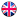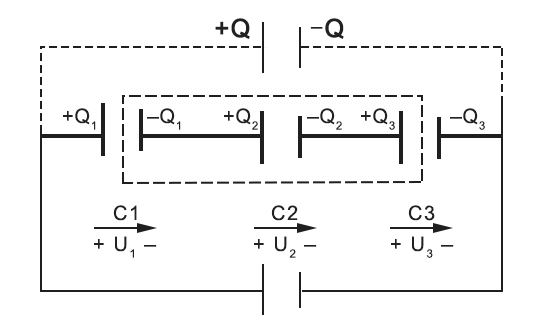EnglishHot keywords :

#### How to Identify Series Capacitors?

2019-08-19

A capacitor is composed of two metal electrodes with an insulating dielectric layer between them. When a voltage is applied between two metal electrodes, the energy is stored on the electrodes, so the capacitor is an energy storage element. Any two conductors that are insulated from each other and very close form a capacitor. The parallel plate capacitor is composed of the polar plate and dielectrics of the capacitor.

Capacitor Characteristics

1. It has charge and discharge characteristics and the ability to prevent DC current from passing through, allowing AC current to pass through.

2. In the process of charging and discharging, the electric charge on the two plates accumulates. That is also the voltage established. Therefore, the voltage on the capacitor can not change abruptly. Capacitor charging: two plates are charged with the same amount of different electric charges, and the absolute value of the charged quantity of each plate is called the charged quantity of the capacitor. Discharge of capacitors: The positive and negative charges of the two poles of capacitors are neutralized through wires. Transient current generation on the wire during discharge.

3. The capacitance reactance of capacitor is inversely proportional to frequency and capacity. That is to say, when the capacitance is analyzed, the frequency and capacity of the signal must be connected.How to Identify Series Capacitors

1. Judging from the characteristics of capacitor connection mode: when two capacitors have only one pole connected to each other and the other pole is not connected to each other.

2. Judging from the polarity of the charged plates of capacitors: capacitors connected by polar plates of different polarities

Characteristic of Series Capacitors

1. The charge of each capacitor is equal: Q1 = Q2 = Q3 = Q

2. The total voltage of series capacitor equals the sum of the voltages on each capacitor: U = U1 + U2 + U33. The reciprocal of the total capacitance of a capacitor bank is equal to the sum of the reciprocal capacitances of each capacitor (the smaller the series is)Calculation Formula for Series CapacitorsSeries capacitors are equivalent to increasing the thickness of dielectrics, that is, increasing the distance between the two plates of capacitors (see figure), thus reducing the capacitance. The capacitance after series connection is calculated by the following formula: